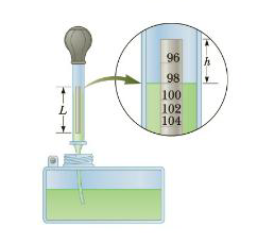Chapter 9, Problem 84AP

Chapter
Section
Textbook Problem

A Hydrometer is an instrument used to determine liquid density. A simple one is sketched in Figure P9.84. The bulb of a syringe is squeezed and released to lift a sample of the liquid of interest into a tube containing a calibrated rod of known density. (Assume the rod is cylindrical.) The rod. of length L and average density ρ0, floats partially immersed in the liquid of density ρ. A length h of the rod protrudes above the surface of the liquid. Show that the density of the liquid is given by ρ = ρ 0 L L   −   hFigure P9.84

To determine
The density of given liquid.

Explanation

The concept of force buoyancy is used for the determination of the density of the liquid.

The weight of the liquid that displaced by the rod is equal to the weight of the rod that is ρVdisplacedg=ρ0Vrodg and the volume of the rod is Vrod=πr2L . The volume of the displaced liquid is equal to the volume of the rod that submerged into the liquid and it is given by Vdisplaced=πr2(Lh)

Still sussing out bartleby?

Check out a sample textbook solution.

See a sample solution

The Solution to Your Study Problems

Bartleby provides explanations to thousands of textbook problems written by our experts, many with advanced degrees!

Get Started